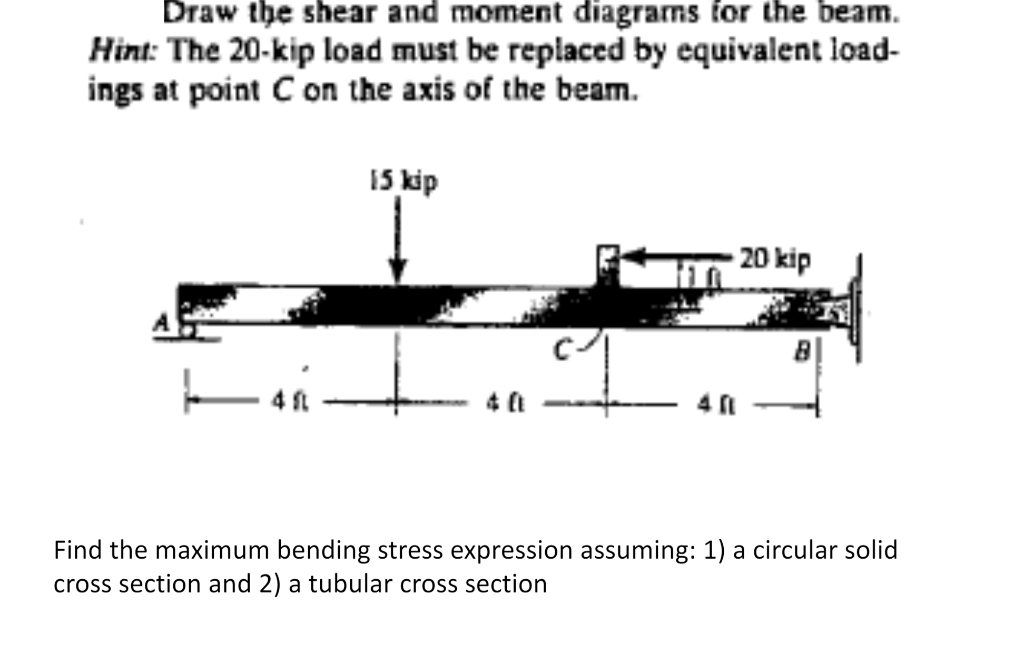# Draw the shear and moment diagrams for the beam. Hint: The 20-kip load must be replaced by equivalent load- ings at point on the axis of the beam. 15 kip 20 kip Find the maximum bending stress expression assuming: 1) a circular solid cross section and 2) a tubular cross section

Question-AnswerCategory: Strength of MaterialsDraw the shear and moment diagrams for the beam. Hint: The 20-kip load must be replaced by equivalent load- ings at point on the axis of the beam. 15 kip 20 kip Find the maximum bending stress expression assuming: 1) a circular solid cross section and 2) a tubular cross section

Draw the shear and moment diagrams for the beam. Hint: The 20-kip load must be replaced by equivalent load- ings at point on the axis of the beam. 15 kip 20 kip Find the maximum bending stress expression assuming: 1) a circular solid cross section and 2) a tubular cross section